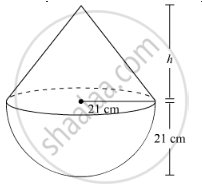Share

# A Toy is in the Form of a Hemisphere Surmounted by a Right Circular Cone of the Same Base Radius as that of the Hemisphere. If the Radius of the Base of the Cone is 21 Cm and Its Volume is 2/3 of the Volume of Hemisphere, Calculate the Height of the Cone and the Surface Area of the Toy. (Use Pi = 22/7) - CBSE Class 10 - Mathematics

ConceptVolume of a Combination of Solids

#### Question

A toy is in the form of a hemisphere surmounted by a right circular cone of the same base radius as that of the hemisphere. If the radius of the base of the cone is 21 cm and its volume is 2/3 of the volume of hemisphere, calculate the height of the cone and the surface area of the toy.
(use pi = 22/7)

#### Solution

Let the height of the conical part be h.

Radius of the cone = Radius of the hemisphere = r = 21 cm

The toy can be diagrammatically represented asVolume of the cone = 1/3pir^2h

Volume of the hemisphere = 2/3pir^3

According to given information:

Volume of the cone =2/3× Volume of the hemisphere

therefore 1/3pir^2h=2/3xx2/3pir^3

⇒h=(2/3xx2/3pir^3)/(1/3pir^2)

⇒h=4/3r

therefore h=4/3xx21cm =28 cm

Thus, surface area of the toy = Curved surface area of cone + Curved surface area of hemisphere

= πrl + 2πr2

=pirsqrt(h^2+r^2)+2pir^2

=pir(sqrt(h^2+r^2)+2r)

=22/7xx21cm(sqrt((28cm)^2+(21cm)^2)+2xx21cm)

=66(sqrt(784+441)+42)cm^2

66(sqrt(1225)+42)cm^2

= 66(35+42) cm2

= 66 x 77 cm2

= 5082 cm2

Is there an error in this question or solution?

#### APPEARS IN

RD Sharma Solution for 10 Mathematics (2018 to Current)
Chapter 14: Surface Areas and Volumes
Ex. 14.20 | Q: 26 | Page no. 62
Solution A Toy is in the Form of a Hemisphere Surmounted by a Right Circular Cone of the Same Base Radius as that of the Hemisphere. If the Radius of the Base of the Cone is 21 Cm and Its Volume is 2/3 of the Volume of Hemisphere, Calculate the Height of the Cone and the Surface Area of the Toy. (Use Pi = 22/7) Concept: Volume of a Combination of Solids.
S